How Cheenta works to ensure student success?
Explore the Back-Story

# I.S.I B.STAT 2018 | SUBJECTIVE -4### PROBLEM

Let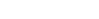be a continous function such that for all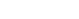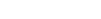Define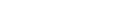foris a constant function

## HINT

Use leibniz rule for differentiation under integral sign

## SOLUTION

using leibniz rule for differentiation under integral sign we get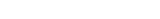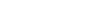[ Because f(3x)=f(x)]
Since the derivative of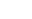is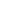for all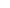, Henceis a constant function

### PROBLEM

Letbe a continous function such that for allDefineforis a constant function

## HINT

Use leibniz rule for differentiation under integral sign

## SOLUTION

using leibniz rule for differentiation under integral sign we get[ Because f(3x)=f(x)]
Since the derivative ofisfor all, Henceis a constant function

This site uses Akismet to reduce spam. Learn how your comment data is processed.

### Knowledge Partner